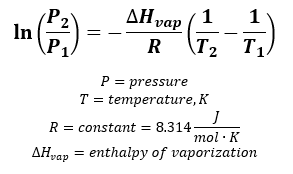# Problem: The enthalpy of vaporization for acetone is 32.0 kJ/mol. The normal boiling point for acetone is 56.5 ˚C. What is the vapor pressure of acetone at 23.5 ˚C?

###### FREE Expert Solution

We need an equation that relates vapor pressure, temperature and enthalpy of vaporization. We're going to use the Clausius-Clapeyron Equation to solve this problem:Given:

Normal boiling point of acetone (T1) = 56.5°C + 273.15 = 329.65 K
Normal pressure (P1) = 1 atm
T2 = 23.5°C + 273.15 = 296.65 K
vapor pressure (P2) = ?
ΔHvap = 32.0 kJ/mol

Substitute values and calculate for P2:

82% (314 ratings)###### Problem Details

The enthalpy of vaporization for acetone is 32.0 kJ/mol. The normal boiling point for acetone is 56.5 ˚C. What is the vapor pressure of acetone at 23.5 ˚C?